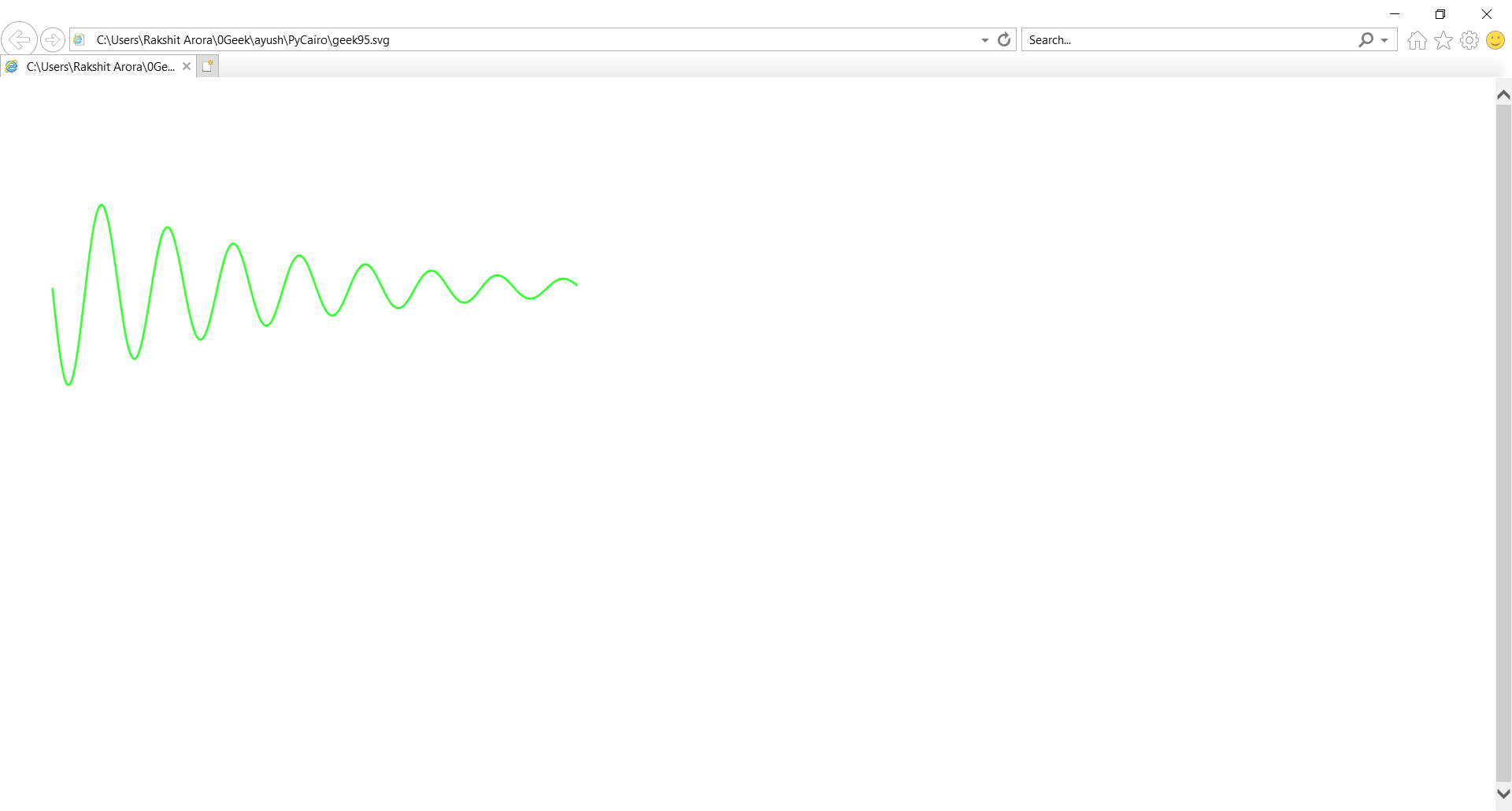# PyCairo- Drawing Function curve

• Last Updated : 12 Nov, 2020

In this article we will learn how we can draw a simple function curve using PyCairo in python. n mathematics, the graph of a function f is the set of ordered pairs, where f(x) = y. In the common case where x and f(x) are real numbers, these pairs are Cartesian coordinates of points in two-dimensional space.

PyCairo : Pycairo is a Python module providing bindings for the cairo graphics library.This library is used for creating SVG i.e vector files in python. The easiest and quickest way to open an SVG file to view it (read only) is with a modern web browser like Chrome, Firefox, Edge, or Internet Explorer—nearly all of them should provide some sort of rendering support for the SVG format.

Attention geek! Strengthen your foundations with the Python Programming Foundation Course and learn the basics.

To begin with, your interview preparations Enhance your Data Structures concepts with the Python DS Course. And to begin with your Machine Learning Journey, join the Machine Learning - Basic Level Course

SVG file is a graphics file that uses a two-dimensional vector graphic format created by the World Wide Web Consortium (W3C). It describes images using a text format that is based on XML. SVG files are developed as a standard format for displaying vector graphics on the web.

Steps of Implementation :

1. Import the Pycairo & Math module.
2. Create a SVG surface object and add context to it.
3. Creating list for creating points for functional curve
4. Traversing and plotting Points
5. Setting color of the context & line width

Below is the Implementation :

## Python3

 `# importing pycairo``import` `cairo``# importing Math to Sin & exp Function``import` `math`` ` `# Variable X``x ``=` `0``# Creating list for creating points``points ``=` `[]``# Creating Loop for points``while` `x < ``5``:``    ``# Creating point corresponding x``    ``y ``=` `math.sin(``10``*``x)``*``math.exp(``-``x``/``2``)``    ``# Adding points to the list``    ``points.append((x``*``100` `+` `50``, y``*``100` `+` `200``))``    ``# Incrementing by 0.01 Variable x``    ``x ``+``=` `0.01`` ` `# creating a SVG surface``# here geek95 is file name & 700, 700 is dimension``with cairo.SVGSurface(``"geek95.svg"``, ``700``, ``700``) as surface:`` ` `    ``# creating a cairo context object for SVG surface``    ``# useing Context method``    ``context ``=` `cairo.Context(surface)`` ` `    ``context.move_to(``*``points[``0``])``    ``# Traversing Points``    ``for` `p ``in` `points[``1``:]:``        ``# Ploting point``        ``context.line_to(``*``p)`` ` `    ``# setting width of the context``    ``context.set_line_width(``2``)``    ``# setting color of the context``    ``context.set_source_rgb(``0.2``, ``1``, ``0.2``)``    ``# stroke out the color and width property``    ``context.stroke()`` ` `# printing message when file is saved``print``(``"File Saved"``)`

Output :My Personal Notes arrow_drop_up